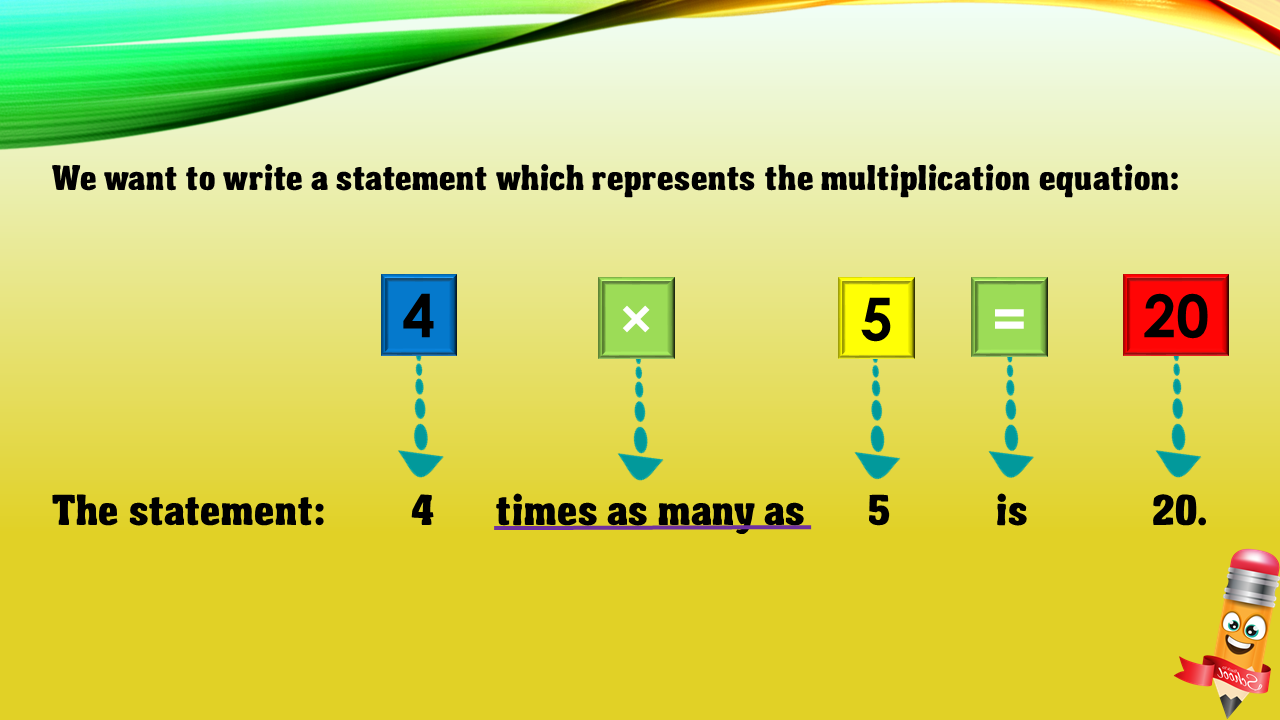1
visibility

Choose one statement which represents the equation:
4 × 5 = 20 ?

• A

4 more than 5 is 20.

• B

5 less than 20 is 4.

• C

4 times as many as 5 is 20.

• D

5 times as many as 20 is 4.